# Creating plots in R using ggplot2 - part 5: scatterplots

written in

This is the fifth tutorial in a series on using ggplot2 I am creating with Mauricio Vargas Sepúlveda. In this tutorial we will demonstrate some of the many options the ggplot2 package has for creating and customising scatterplots. We will use R’s airquality dataset in the datasets package. In order to reduce the complexity of these data a little, we will only be looking at the final three months in the dataset (July, August and September).

Mauricio and I have also published these graphing posts as a book on Leanpub. We tend to put any changes or updates to the code in the book before these blog posts, so please check it out if you have any issues with the code examples in this post; otherwise feel free to contact us with any questions!

In this tutorial, we will work towards creating the scatterplot below. We will take you from a basic scatterplot and explain all the customisations we add to the code step-by-step.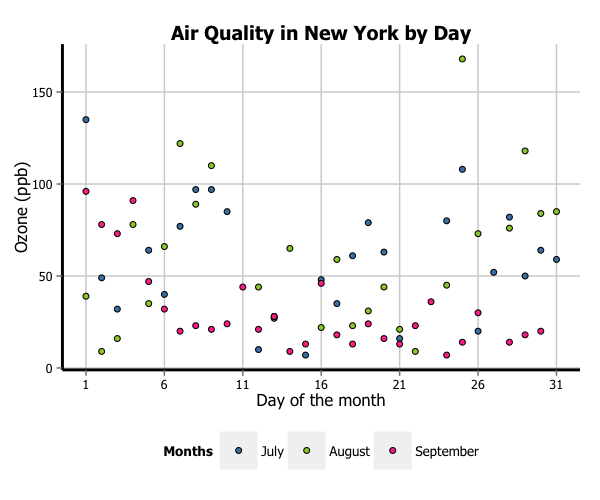The first thing to do is load in the data, as below:

library(ggplot2)
library(ggthemes)
library(extrafont)
library(datasets)
data(airquality)


We will then trim the data down to the final three months and turn the Month variable into a labelled factor variable. We end up with a new dataset called aq_trim.

aq_trim <- airquality[which(airquality$Month == 7 | airquality$Month == 8 |
airquality$Month == 9), ] aq_trim$Month <- factor(aq_trim\$Month,
labels = c("July", "August", "September"))


### Basic scatterplot

In order to initialise a scatterplot we tell ggplot that aq_trim is our data, and specify that our x-axis plots the Day variable and our y-axis plots the Ozone variable. We then instruct ggplot to render this as a scatterplot by adding the geom_point() option.

p5 <- ggplot(aq_trim, aes(x = Day, y = Ozone)) +
geom_point()
p5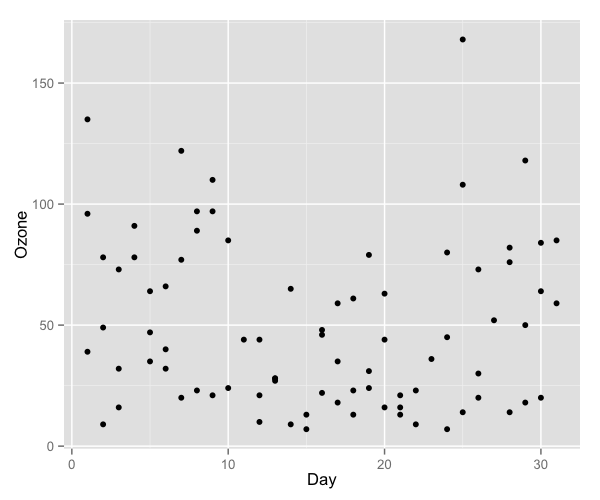### Changing the shape of the data points

Perhaps we want the data points to be a different shape than a solid circle. We can change these by adding the shape argument to geom_point. An explanation of the allowed arguments for shape are described in this article. In this case, we will use shape 21, which is a circle that allows different colours for the outline and fill.

p5 <- ggplot(aq_trim, aes(x = Day, y = Ozone)) + geom_point(shape = 21)
p5### Adjusting the axis scales

To change the x-axis tick marks, we use the scale_x_continuous option. Similarly, to change the y-axis we use the scale_y_continuous option. Here we will change the x-axis to every 5 days, rather than 10, and change the range from 1 to 31 (as 0 is not a valid value for this variable).

p5 <- p5 + scale_x_continuous(breaks = seq(1, 31, 5))
p5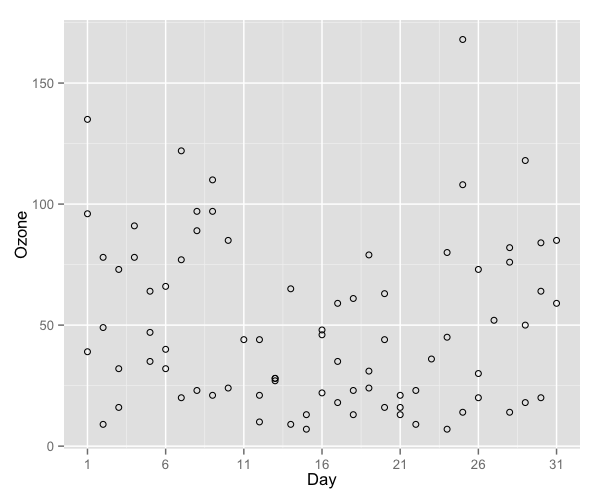### Adjusting axis labels & adding title

To add a title, we include the option ggtitle and include the name of the graph as a string argument. To change the axis names we add x and y arguments to the labs command.

p5 <- p5 + ggtitle("Air Quality in New York by Day") +
labs(x = "Day of the month", y = "Ozone (ppb)")
p5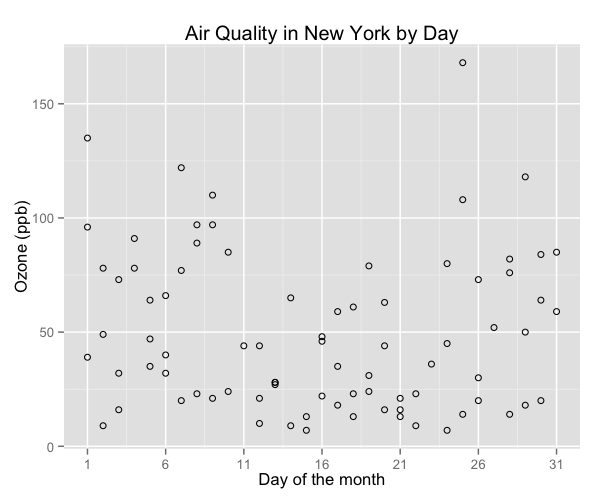### Adjusting the colour palette

There are a few options for adjusting the colour. The most simple is to make every point one fixed colour. You can reference colours by name, with the full list of colours recognised by R here. Let’s try making the outline mediumvioletred and the fill springgreen.

p5 <- ggplot(aq_trim, aes(x = Day, y = Ozone)) +
geom_point(shape = 21, colour = "mediumvioletred",
fill = "springgreen") +
ggtitle("Air Quality in New York by Day") +
labs(x = "Day of the month", y = "Ozone (ppb)") +
scale_x_continuous(breaks = seq(1, 31, 5))
p5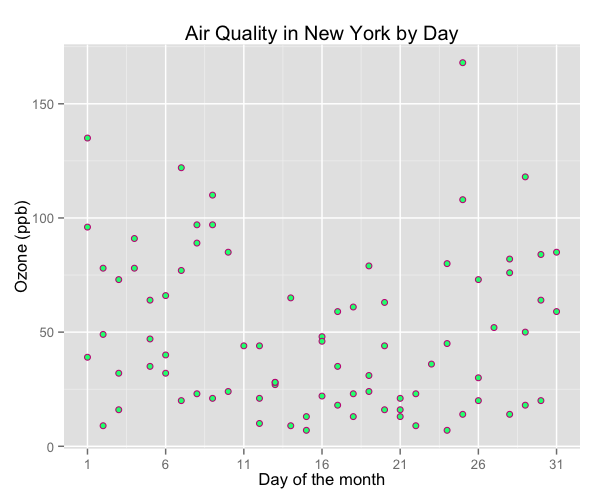You can change the colours using specific HEX codes instead. Here we have made the outline #000000 (black) and the fill “#40b8d0 (vivid cyan).

p5 <- ggplot(aq_trim, aes(x = Day, y = Ozone)) +
geom_point(shape = 21, colour = "#000000", fill = "#40b8d0") +
ggtitle("Air Quality in New York by Day") +
labs(x = "Day of the month", y = "Ozone (ppb)") +
scale_x_continuous(breaks = seq(1, 31, 5))
p5You can also change the colour of the data points according to the levels of another variable. This can be done either as a continuous gradient, or as a levels of a factor variable. Let’s change the colour by the values of temperature:

p5 <- ggplot(aq_trim, aes(x = Day, y = Ozone, fill = Temp)) +
geom_point(shape = 21) +
ggtitle("Air Quality in New York by Day") +
labs(x = "Day of the month", y = "Ozone (ppb)") +
scale_x_continuous(breaks = seq(1, 31, 5))
p5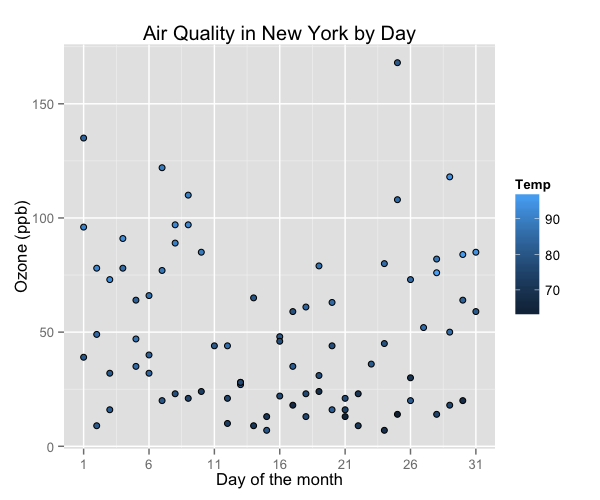We can change the gradient’s colours by adding the scale_fill_continuous option. The low and high arguments specify the range of colours the gradient should transition between.

p5 <-  p5 + scale_fill_continuous(low = "plum1", high = "purple4")
p5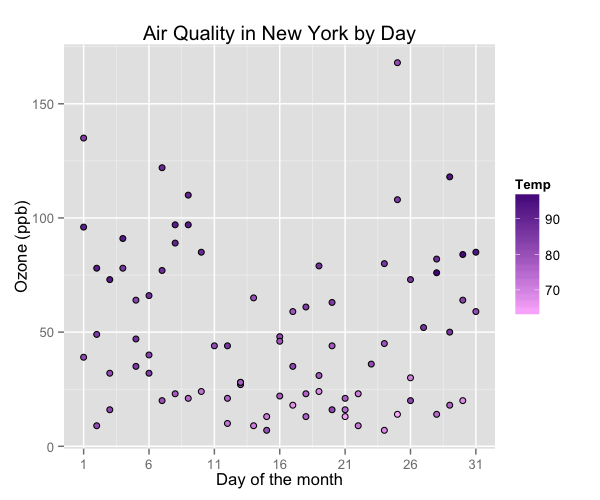We can see that higher temperatures seem to have higher ozone levels.

Let’s now change the colours of the data points by a factor variable, Month.

p5 <- ggplot(aq_trim, aes(x = Day, y = Ozone, fill = Month)) +
geom_point(shape = 21) +
ggtitle("Air Quality in New York by Day") +
labs(x = "Day of the month", y = "Ozone (ppb)") +
scale_x_continuous(breaks = seq(1, 31, 5))
p5Again, we can change the colours of these data points, this time using scale_fill_manual.

fill = c("steelblue", "yellowgreen", "violetred1")

p5 <- p5 + scale_fill_manual(values = fill)
p5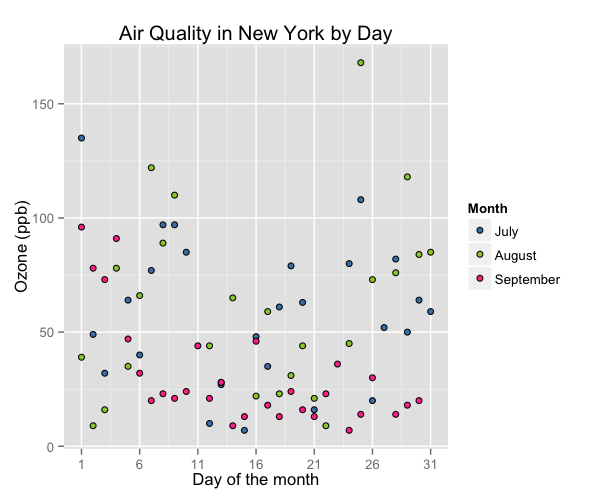### Adjusting legend position

To adjust the position of the legend from the default spot of right of the graph, we add the theme option and specify the legend.position = "bottom" argument. We can also change the legend shape using the legend.direction = "horizontal" argument.

p5 <- p5 + theme(legend.position = "bottom", legend.direction = "horizontal")
p5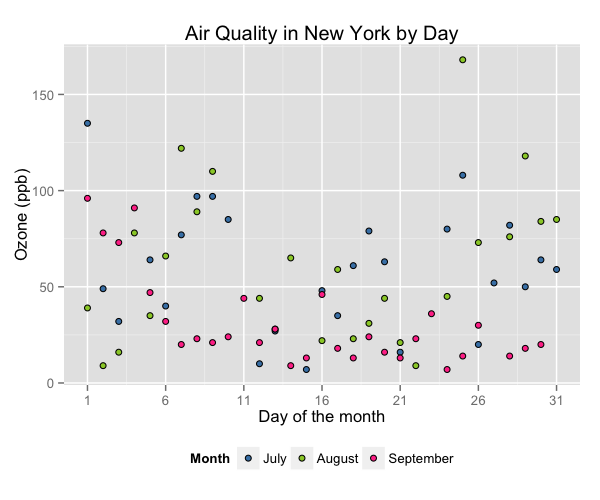### Using the white theme

As explained in the previous posts, we can also change the overall look of the plot using themes. We’ll start using a simple theme customisation by adding theme_bw() after ggplot(). As you can see, we can further tweak the graph using the theme option, which we’ve used so far to change the legend.

p5 <- ggplot(aq_trim, aes(x = Day, y = Ozone, fill = Month)) +
geom_point(shape = 21) +
theme_bw() +
theme() +
ggtitle("Air Quality in New York by Day") +
labs(x = "Day of the month", y = "Ozone (ppb)", fill = "Months") +
scale_x_continuous(breaks = seq(1, 31, 5)) +
scale_fill_manual(values = fill) +
scale_size(range = c(1, 10)) +
theme(legend.position="bottom", legend.direction="horizontal")
p5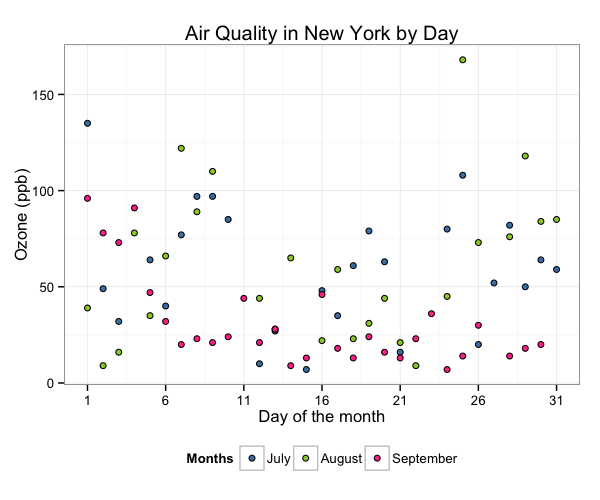### Creating an XKCD style chart

Of course, you may want to create your own themes as well. ggplot2 allows for a very high degree of customisation, including allowing you to use imported fonts. Below is an example of a theme Mauricio was able to create which mimics the visual style of XKCD. In order to create this chart, you first need to import the XKCD font, install it on your machine and load it into R using the extrafont package. These instructions are taken from here:

library(extrafont)

dest="xkcd.ttf", mode="wb")
system("mkdir ~/.fonts")
system("cp xkcd.ttf  ~/.fonts")
font_import(paths = "~/.fonts", pattern="[X/x]kcd")
fonts()


You can then create your graph:

fill <- c("#56B4E9", "#F0E442", "violetred1")

p5 <- ggplot(aq_trim, aes(x = Day, y = Ozone, fill = Month)) +
geom_point(shape = 21) +
ggtitle("Air Quality in New York by Day") +
labs(x = "Day of the month", y = "Ozone (ppb)", fill = "Months") +
scale_x_continuous(breaks = seq(1, 31, 5)) +
scale_fill_manual(values = fill) +
scale_size(range = c(1, 10)) +
theme(legend.position="bottom", legend.direction="horizontal",
axis.line = element_line(size=1, colour = "black"),
panel.grid.major = element_blank(),
panel.grid.minor = element_blank(),
panel.border = element_blank(),
panel.background = element_blank(),
plot.title=element_text(family="xkcd-Regular"),
text=element_text(family="xkcd-Regular"),
axis.text.x=element_text(colour="black", size = 10),
axis.text.y=element_text(colour="black", size = 10))
p5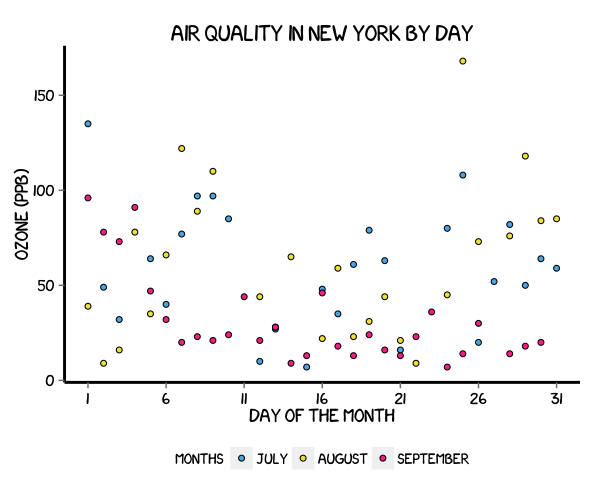### Using ‘The Economist’ theme

There are a wider range of pre-built themes available as part of the ggthemes package (more information on these here). Below we’ve applied theme_economist(), which approximates graphs in the Economist magazine.

p5 <- ggplot(aq_trim, aes(x = Day, y = Ozone, fill = Month)) +
theme_economist() +
scale_fill_economist() +
geom_point(shape = 21) +
ggtitle("Air Quality in New York by Day") +
labs(x = "Day of the month", y = "Ozone (ppb)", fill = "Months") +
scale_x_continuous(breaks = seq(1, 31, 5)) +
scale_size(range = c(1, 10)) +
theme(legend.position = "bottom", legend.direction = "horizontal",
plot.title = element_text(family="Tahoma"),
text = element_text(family = "Tahoma"),
axis.title = element_text(size = 12),
legend.text = element_text(size = 9),
legend.title=element_text(face = "bold", size = 9))
p5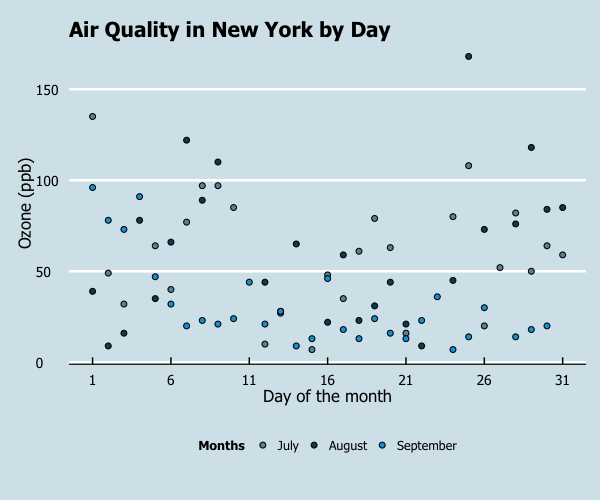### Creating your own theme

As before, you can modify your plots a lot as ggplot2 allows many customisations. Here we present our original result shown at the top of page.

fill = c("steelblue", "yellowgreen", "violetred1")

p5 <- ggplot(aq_trim, aes(x = Day, y = Ozone, fill = Month)) +
geom_point(shape = 21) +
ggtitle("Air Quality in New York by Day") +
labs(x = "Day of the month", y = "Ozone (ppb)", fill = "Months") +
scale_x_continuous(breaks = seq(1, 31, 5)) +
scale_size(range = c(1, 10)) +
scale_fill_manual(values = fill) +
theme(legend.position = "bottom", legend.direction = "horizontal",
axis.line = element_line(size=1, colour = "black"),
panel.grid.major = element_line(colour = "#d3d3d3"),
panel.grid.minor = element_blank(),
panel.border = element_blank(), panel.background = element_blank(),
plot.title = element_text(size = 14, family = "Tahoma", face = "bold"),
text=element_text(family="Tahoma"),
axis.text.x=element_text(colour="black", size = 9),
axis.text.y=element_text(colour="black", size = 9))
p5